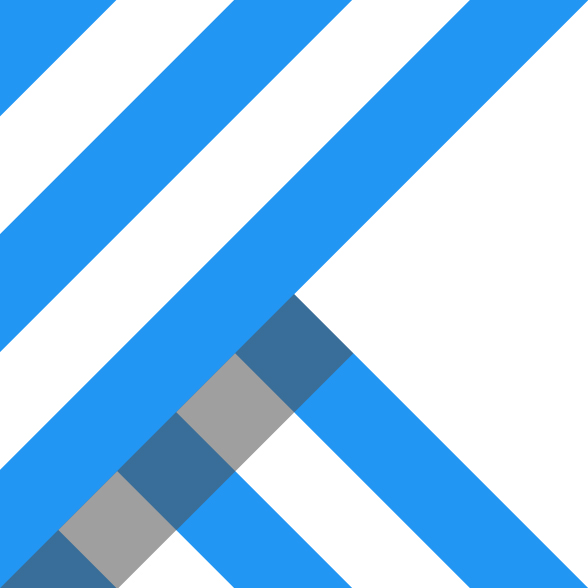komputation - Komputation is a neural network framework for the Java Virtual Machine written in Kotlin and CUDA C. - Kotlin Resources# Komputation

Komputation is a neural network framework for the Java Virtual Machine written in Kotlin and CUDA C.

## Maven

Komputation is available through Maven Central:

``````<dependency>
<groupId>com.komputation</groupId>
<artifactId>komputation</artifactId>
<version>0.12.5</version>
</dependency>
``````

## Layers

• Entry points:
• Standard feed-forward networks:
• Convolutional neural networks (CNNs):
• Recurrent neural networks:
• Dropout

• Activation functions:
• Other layers:

## GPU/CUDA demos

• Boolean functions:
• Word embedding toy problem:
• Total:
• Increment:
• MNIST:
• TREC:

## Sample code

The following code instantiates a GPU-accelerated convolutional neural network for sentence classification:

``````    val sentenceClassifier = cudaNetwork(
batchSize,
lookup(embeddings, maximumDocumentLength, embeddingDimension, optimization),
convolution(numberFilters, filterWidth, filterHeight, initialization, optimization),
relu(),
dropout(random, keepProbability),
dense(numberCategories, Activation.Softmax, initialization, optimization)
)
``````

See the TREC demo for more details.

## Optimization

`compile "com.komputation:komputation:0.12.5"`

## Related Libraries

#### komputation

Komputation is a neural network framework for the Java Virtual Machine written in Kotlin and CUDA C.

Last updated 3 mins ago